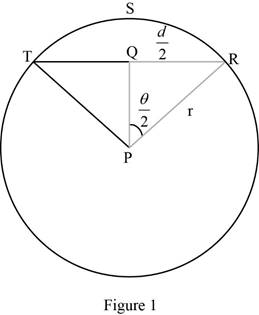# The value of lim θ → 0 + s d .### Single Variable Calculus: Concepts...

4th Edition
James Stewart
Publisher: Cengage Learning
ISBN: 9781337687805### Single Variable Calculus: Concepts...

4th Edition
James Stewart
Publisher: Cengage Learning
ISBN: 9781337687805

#### Solutions

Chapter 3.3, Problem 49E
To determine

## To find: The value of limθ→0+sd.

Expert Solution

The value of limθ0+sd=1.

### Explanation of Solution

Given:

The circular arc of length is s and the chord of length is d.

Formula used:

Circular arc length: s = rθ, where r is the radius of the circle.

Result used:

The value of limit limx0sinxx=1.

Calculation:

The given picture is shown in the below Figure 1.From Figure 1, TQ=QR=d2 and RPQ=θ2.

Calculate the value of d.

From the triangle PQR as shown in Figure 1,

sinθ2=opposite sidehypotenusesinθ2=d2rrsinθ2=d22rsinθ2=d

Therefore, the value is d=2rsinθ2.

Consider limθ0+sd.

Substitute s=rθ and d=2rsinθ2

limθ0+sd=limθ0+rθ2rsinθ2=limθ0+θ2sinθ2

Let x=θ2 then x will approach to 0+ as θ approaches to 0+.

limθ0+sd=limx0+xsinx=limx0+1sinxx=1limx0+sinxx

Use Result stated above and obtain the value of the limit.

limθ0+sd=11=1

Therefore, the value of limθ0+sd=1.

### Have a homework question?

Subscribe to bartleby learn! Ask subject matter experts 30 homework questions each month. Plus, you’ll have access to millions of step-by-step textbook answers!